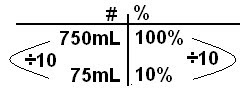## Tuesday, December 21, 2010

### Ryan's Scribe Post

8. The original price of a jacket was \$84.00. A store manager marked the price down by 25 1/2 %. By how much was the price reduced?
Math Info
*
Original Price = \$84. 00
* Price down by 25 1/2%
* 25 1/2% = 25. 5%
Math Info
* 25. 5% = \$21. 42
The price was reduced by \$21. 42.
10. When water freezes, its volume increases by approximately 10%.
a) By how much does the volume of 750mL of water increase when it freezes?
Math Info
* 10% increaseMath Info
* 10% = 75mL
The volume increases by 75mL when it has an original volume of 750mL.
b) What is the volume of ice created?
Math Info
* 750mL = 100%
* 10% = 75mL
750mL + 75mL = 825mL
The volume is 825mL.
16. Josephine scored 12 baskets out of 30 shots in her first basketball game this year. Her scoring average was then 40%. The next game, she made ten shots and raised her scoring average for both games to 50%. How many of the ten shots in the second game were baskets?
Math Info
First Game
* Took 30 shots
Second Game
* Took 10 shots
* __ shots out of 10 = baskets
* Average = 40%
All Games
* Scoring Averge = 50%
* Took 40 shots [30 + 10]
* 50% of 40 shots = 20 shots

Math Info
* 8 shots out of 10 = baskets
1.